# Intersections (1)

The boolean operations are probably the most interesting parts of the Boost.Geometry library.

Boolean operations are, based on two polygons:
• intersection, returning polygons containing areas present in both input polygons
• union, returning polygons containing all areas present in either one of the input polygons
• difference, returning polygons containing areas present in one of the input polygons, but not in the other
• symmetric difference, returning polygons containing areas present in one of either input polygons, but not in both
In other words, boolean operations are spatial implementations of mathematical set theoretic operations. Based on two polygons A and B:
• intersection: A and B, also written as A ∩ B
• union: A or B, also written as A ∪ B
• difference: A and not B, also written as A \ B
• symmetric difference: A xor B, also written as A ∆ B
The difference operation (also known as relative complement) is the only operation of these which is not commutative, A \ B results in another geometry than B \ A.

## The basics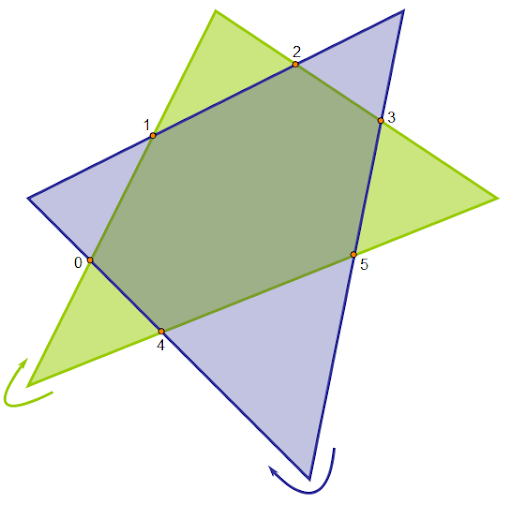This blog will not explain the whole algorithm, that would be way too long. Other of my future blogs or a future article will explain more details. The algorithm used in Boost.Geometry is an adapted version of the Weiler-Atherton algorithm. The basics are quite simple, and explained here, and also in adapted versions as from Greiner-Hormann. Note that I have adapted the algorithm myself, such that intersection points are not inserted in the originals but stored separately. Which is essential because we don't want to change the input polygons, and we neither want to copy the input polygons. I have made more adaptations, such that all situations including self-tangencies (in some articles called degenerations) and robustness are handled.

So only the very basics in this blog. There are two input polygons, A and B (here: green and blue), both oriented clockwise. The arrows indicate orientation at the starting points. Intersection points (orange) are calculated. During the calculation of the intersection points, traversal information is gathered: for an intersection (usually: go to the right), would it continue following the green or the blue outline... For example, at point 0, above, it is figured out that for an intersection the green line should be followed, and for a union the blue line. Both until the next intersection point is encountered. That requires sorting which is used heavily within the algorithm.

Turning right for intersections or left for unions is also visualized below, from an older part of the GGL-doc: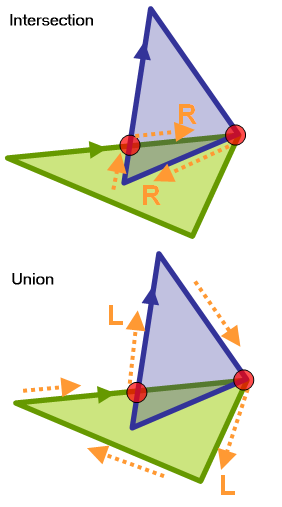How traversal information is figured out, and how intersection points are sorted, will be explained in another blog, maybe much later.

So intersection points and turn information are basically all that is necessary up to this point. The traverse function uses this information, and adds points to the accumulated output polygon. Added points can be either intersection points or original vertices. The output intersection then looks like this (slightly shifted by hand to show it better):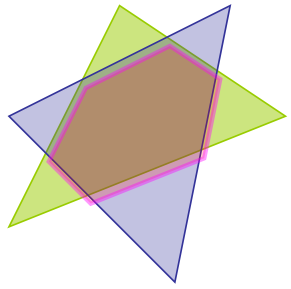Note that it can become (often much) more complicated, holes, intersections at vertices, self-tangencies, polygons-within-holes, multi-polygons, etc.

Here the process for the intersection of a polygon with a polygon with two holes: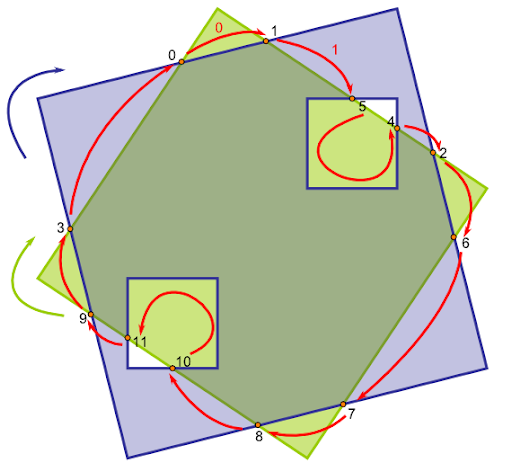Note that the numbers of the intersection points are shown in the order found, and not in the order of traversal. It is not possible to indicate intersection points with an order of traversal, because that order differs for intersection and union (check this in the picture above). The (manually added) red arrows depict the traversal for intersection, starting with the red "0".

Note also that holes are not a big addition to the algorithm, if they are oriented counterclockwise in clockwise polygons (and vice versa). This is an assumption that is made within Boost.Geometry. If this is the case, traversal can take its routes always in the same way. The union operation would create two holes, which are different from the original holes and stored automatically in the correct orientation.

## All boolean algorithms are the same

I mean to say here: intersection, union, and (symmetric) difference are all the same.

We have seen above that for intersection and union the same information is gathered. Only one variable, the traversal-direction, determines what is the output. So easy, we have one algorithm in which we calculate intersection or union.

Now for the difference, it turns out that if we reverse one of the polygons (e.g. green one), such that it is oriented counter-clockwise, the difference is calculated automatically by calculating the intersection of that reversed A and the still normal B. That is not that strange, because it follows from a mathematical rule. The difference of A and B is the same as the intersection of the complement of A with B. And the complement of A (Ac) is just its reverse. Voila, we do another function with the same algorithm.

The complement (from older GGL-doc) of polygon A is the whole world but A.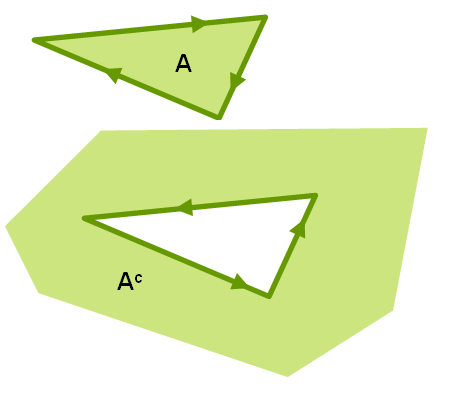The symmetric difference, A xor B, is just adding the two differences: it is also defined as A and not B unioned by B and not A.

Concluding this: all operations intersection, union, difference and symmetric difference are calculated with this one algorithm. The algorithm consists of several sub algorithms, relevant for all operations, and I hope to describe them later in more detail.

## Reversing

How do we take the reverse in the algorithm? In the past, I cheated and reversed the polygon before doing the algorithm. That was easy, but has two drawbacks: 1) the input must be copyable and 2) it takes time to reverse. So we don't do this anymore. We just iterate through it in reverse way. That is easy enough, using an iterator walking reversely though the vertices. Or, in the case of Boost.Geometry, we use a reversible_range, as explained in previous blog. It is all implemented using specializations and meta-programming.

The difference is shown below. On the left side the result, again shifted slightly to make things more clear. The right side shows the same but in a different way: the intersection of the complement of A (green) with B (blue).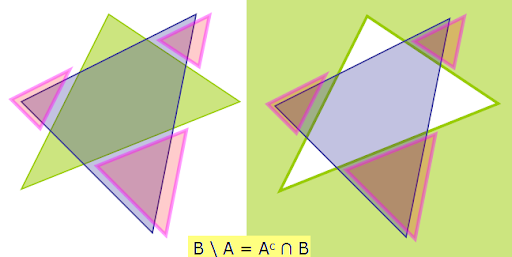## The one algorithm handles all geometries

We have seen above that one algorithm is enough and that we can walk in reverse through the polygons to support differences. What if we have counterclockwise polygons? The answer is the same, we walk through it using our reversible ranges, which are in fact Boost.Range reverse_range adaptors. So we calk backwards through it during getting intersection points, and later when vertices are necessary for the output, and actually everywhere where it is used.

So we can handle clockwise and counterclockwise input polygons, and we can even handle one clockwise, one counterclockwise, and the output can also be clockwise or counterclockwise at wish. That last feature is done by appending points during traversal to the back of the output rings, or inserting them at the front.

The algorithm also handles combinations of rectangles and polygons, because a rectangle can be seen as a polygon. The only difference is that the calculation of intersection points is done more efficient. Furthermore, multi-polygons can be handled. Note that the intersection of two polygons can result in a multi-polygon.

So this seems a litany of what the algorithm can handle, and it does not stop here. Because Boost.Geometry algorithms are independant on their input point types, and also independant on their input polygon types, and other geometry types, the algorithm can handle any geometry type based on any point type. So it can handle 4-byte-floats but also 8-byte-doubles or high-precision types like TTMath. It can handle boost::geometry polygons but also ESRI polygons, provided those polygons are adapted to Boost.Geometry.

Another aspect of this algorithm is that it is coordinate system agnostic. It can handle cartesian polygons, but can also calculate intersections of spherical polygons. This is possible because the algorithm is based on sub-algorithms as calculating the intersection points, and finding the side (left/right) of a point with respect to two other points. If those algorithms are provided (currently they are not...) the intersection algorithm will handle them.

## The fastest...

Finally, the implementation of boolean operations in Boost.Geometry is also the most efficient intersection algorithm which can be found. If people don't believe this, or want to test it, or help benchmarking: there are Open Source benchmarks. Other people are more independant as I am, help would be welcome.

Below intersection of two multi-polygons with self-tangencies, intersection is again shifted slightly. This comes from one of the robustness tests.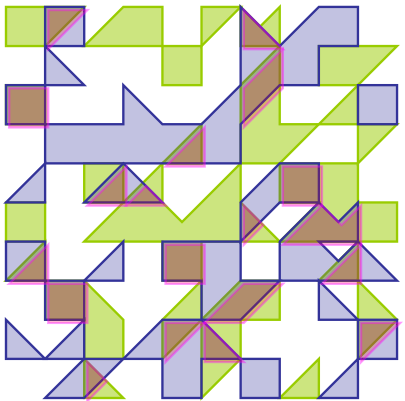## Final remarks

Using the reversible_range for (symmetric) difference instead of reversing geometries, in the implementaiton,  was done yesterday. So all this stuff above was planned but, until yesterday, not implemented like it is today. So another step forwards is made.

The review report (from now more than a year ago) said:

Boolean operations: while the library provides a set of Boolean operations those seem to be not complete and/or robust in terms of clockwise/counterclockwise geometries, closed/open polygons. Robust Boolean operations are a strong requirement, so this should be fixed as reported by at least one reviewer.

This point is now dealt with. There are still a few tweaks to be done but they will disappear coming weeks. I didn't discuss robustness in this blog, and open polygons neither, but these issues are already addressed too.

Don't think you can implement boolean operations easily, though the basic algorithm is quite simple... There are about 100 cases for extraction of turn information from two intersecting segments. Besides that there are also dozens of cases necessary for self-tangencies, in GIS not so ubiquitous, but in games certainly important. I started the algorithm in January 2008 and rewrote it two times after that, one time before the review, and another time after it because there were issues with the implementation, self-tangencies, and robustness. Now it should be ready for production.

The implementation is heavily based on C++, generic programming, templates, specialization and meta-programming. I don't think it is easily translatable into another language (but D). Also in C#, where specializations (at runtime) are possible according to one of my previous blogs, metaprogramming is not possible, it is a compile-time mechanism.

1.you say that the algorithm is 'coordinate system agnostic. It can handle cartesian polygons, but can also calculate intersections of spherical polygons'.

IIRC, when I investigated the matter, I found out that the code will handle polygons expressed in spherical coordinates, but not spherical poligons, which really are sequences of great circle segments and methematically more demanding. Merely using a different coordinate system to express the same sequnce of lines connecting points is trivial.

Please correct me if I'm mistaken

2.The algorithm itself can handle spherical polygons. I know they are sequences of great circle segments.

As soon as segment-intersections of great circles are there, and side-information (which is already there), the polygon intersection routine itself should be able to create correct intersections, unions and differences.

1.Is the segment-intersection piece you mention (for spherical polygon intersections) in place yet?

2.My attempts to compile union_ and intersect operations for spherical polygons failed (see code below), so it seems that the necessary pieces aren't added yet, although I may be doing something wrong. When CARTESIAN=1 it compiles and standard functional examples work well. I'm using boost 1.50 (BOOST_LIB_VERSION = "1_50").

Both the union_ and intersect examples fail to compile with a long chain of errors ending with the following:

/opt/local/include/boost/mpl/assert.hpp:79:5: Candidate function [with C = false] not viable: no known conversion from 'boost::mpl::failed ************(boost::geometry::strategy::side::services::default_strategy::NOT_IMPLEMENTED_FOR_THIS_TYPE::************)(types)' to 'typename assert::type' (aka 'mpl_::assert') for 1st argument;

namespace bg = boost::geometry;
namespace bgm = boost::geometry::model;
#define CARTESIAN 0
#if CARTESIAN
typedef bgm::point PointType; // bgm::d2::point_xy
#else
typedef bgm::point > PointType;
#endif
typedef bgm::ring RingType;
typedef bgm::polygon PolygonType;
typedef bgm::multi_polygon< PolygonType > MultiPolygonType;

void UnionCompilerTest()
{
PolygonType poly;
MultiPolygonType shape, tmp_union;
boost::geometry::union_( shape, poly, tmp_union ); // compiler test
}

void IntersectionCompilerTest()
{
PolygonType poly1, poly2;
std::deque overlap;
boost::geometry::intersection(poly1, poly2, overlap);
}

3.Indeed, the intersection/union are not yet ready for spherical polygons. The error message you get indicates that the side strategy is not yet there. Besides that the segment-intersection is also not yet there, and there is more.

Thanks for your interest and hopefully I get the time to work on this in the near future.

3.I have encountered a case where the _union algorithm fails at the point where it appears to be testing to see if one of the polygons is self-intersecting. I am currently using boost 1.47. Given the description of the tolerance for self-intersection and self-tangency does this make sense?

4.Please provide the sample-program such that I can see what is wrong and where.
You can mail it to the mailing list on Boost.Geometry at Boost, or mail it to me privately.
Thanks.

5.Hello Barend, I'm trying to find whether a line segment intersects any edge or is completely contained by a polygon. What would you advise. Disjoint and Intersects both give compilation errors so I'm a bit lost!

6.Hi, please try the newest version (from github, develop branch https://github.com/boostorg/geometry/tree/develop ) first. A lot of work has been done on this last months and I think this is solved now.

1.Hi Barend,

Thanks a ton for the response, actually I got it working using a linestring and a polygon. I have the version 1.54 I think. When I passed the linestring in as the second parameter to intersection, there was a compiler error, but when I pass the LineString as the first parameter and the Polygon as the second parameter it seems to work fine! Some documentation on that might help. Are there any plans of enabling 3D support for the disjoint/intersection etc functions? Right now I need to convert my 3D objects to 2D objects manually for almost everything except lines. It would be great to have some easy to use 3D support in Boost.